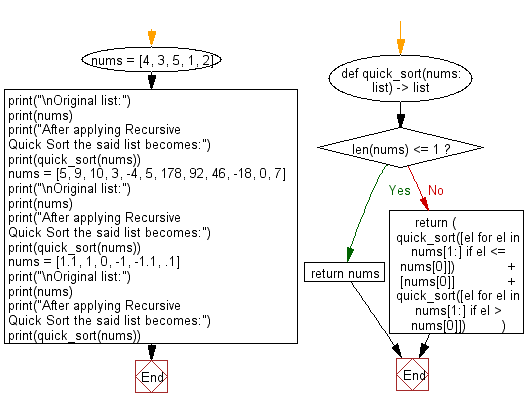﻿ Python: Sort unsorted numbers using Recursive Quick Sort - w3resource# Python: Sort unsorted numbers using Recursive Quick Sort

## Python Search and Sorting : Exercise-28 with Solution

Write a Python program to sort unsorted numbers using Recursive Quick Sort.

Quicksort is a divide and conquer algorithm. It first divides the input array into two smaller sub-arrays: the low elements and the high elements. It then recursively sorts the sub-arrays.

Sample Solution:

Python Code:

``````def quick_sort(nums: list) -> list:
if len(nums) <= 1:
return nums
else:
return (
quick_sort([el for el in nums[1:] if el <= nums])
+ [nums]
+ quick_sort([el for el in nums[1:] if el > nums])
)
nums = [4, 3, 5, 1, 2]
print("\nOriginal list:")
print(nums)
print("After applying Recursive Quick Sort the said list becomes:")
print(quick_sort(nums))
nums = [5, 9, 10, 3, -4, 5, 178, 92, 46, -18, 0, 7]
print("\nOriginal list:")
print(nums)
print("After applying Recursive Quick Sort the said list becomes:")
print(quick_sort(nums))
nums = [1.1, 1, 0, -1, -1.1, .1]
print("\nOriginal list:")
print(nums)
print("After applying Recursive Quick Sort the said list becomes:")
print(quick_sort(nums))
```
```

Sample Output:

```Original list:
[4, 3, 5, 1, 2]
After applying Recursive Quick Sort the said list becomes:
[1, 2, 3, 4, 5]

Original list:
[5, 9, 10, 3, -4, 5, 178, 92, 46, -18, 0, 7]
After applying Recursive Quick Sort the said list becomes:
[-18, -4, 0, 3, 5, 5, 7, 9, 10, 46, 92, 178]

Original list:
[1.1, 1, 0, -1, -1.1, 0.1]
After applying Recursive Quick Sort the said list becomes:
[-1.1, -1, 0, 0.1, 1, 1.1]
```

Flowchart:Python Code Editor:

What is the difficulty level of this exercise?

Test your Programming skills with w3resource's quiz.

﻿

## Python: Tips of the Day

Use Reversed() In for Loops:

```>>> tasks = ['laundry', 'picking up kids', 'gardening', 'cooking']